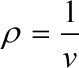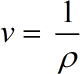Request a Tool

Specific Volume Calculator

Specific volume refers to the volume that is occupied by a unit of mass of a material or substance, also termed as property of materials.

Density
0

Formula• ρ = Density
• v = Specific Volume

Defination / Uses

The reciprocal of the density is the specific volume. The letter "v" stands for it. In a nutshell, specific volume is defined as the volume of a material per unit mass. This online Specific Volume Calculator is useful in calculating the specific volume of a mass unit of substance.

Specific Volume
0

Formula• v = Specific Volume
• ρ = Density

Defination / Uses

The reciprocal of the density is the specific volume. The letter "v" stands for it. In a nutshell, specific volume is defined as the volume of a material per unit mass. This online Specific Volume Calculator is useful in calculating the specific volume of a mass unit of substance.

How to use this calcultor?

There are only two basic steps.

• First select the option from dropdown. What you need to calculate.
• Then add the otherone value in its corresponding field.

Thats it! You will get your answer in less than a second.

The specific volume is obtained by dividing the mass by the volume. It’s also equal to 1/density. You'll need to know the volume (V) and the mass (M) to determine specific volume (m). Volume is usually expressed in cubic metres (m3), whereas mass is expressed in kilos. The specific volume is then determined by dividing the volume by the mass. No sign-up, registration OR captcha is required to use this tool.